## RS Aggarwal Class 8 Solutions Chapter 11 Compound Interest Ex 11B

These Solutions are part of RS Aggarwal Solutions Class 8. Here we have given RS Aggarwal Solutions Class 8 Chapter 11 Compound Interest Ex 11B.

Other Exercises

By using the formula, find the amount and compound interest on :

Question 1.
Solution:
Principal (P) = Rs. 6000
Rate (R) = 9% p.a.
Period (n) = 2 years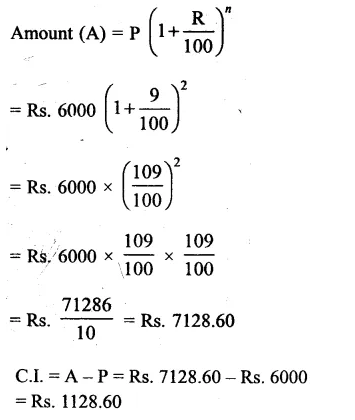Question 2.
Solution:
Principal (P) = Rs. 10000
Rate (R) = 11% p.a.
Period (n) = 2 years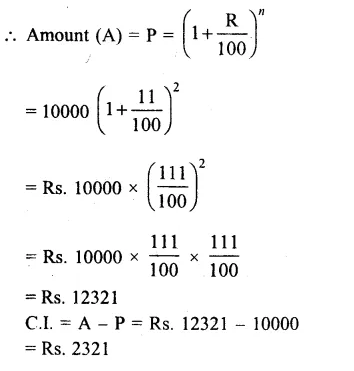Question 3.
Solution:
Principal (P) = Rs. 31250
Rate (R) = 8% p.a.
Period (n) = 3 years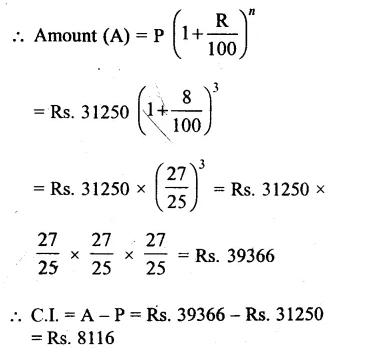Question 4.
Solution:
Principal (P) = Rs. 10240
Rate (R) = $$12\frac { 1 }{ 2 }$$% = $$\\ \frac { 25 }{ 2 }$$% p.a.
Period (n) = 3 years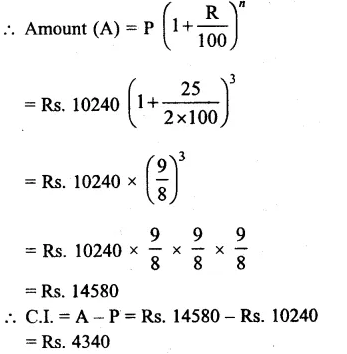Question 5.
Solution:
Principal (P) = Rs. 62500
Rate (R) = 12% p.a.
Period (n) = 2 years 6 months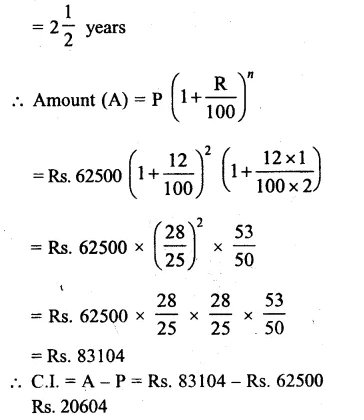Question 6.
Solution:
Principal (P) = Rs. 9000
Rate (R) = 10% p.a.
Period (n) = 2 years 4 months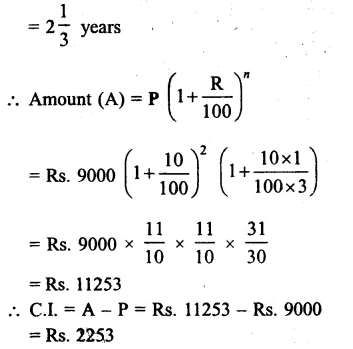Question 7.
Solution:
Principal (P) = Rs. 8000
Period (n) = 2 years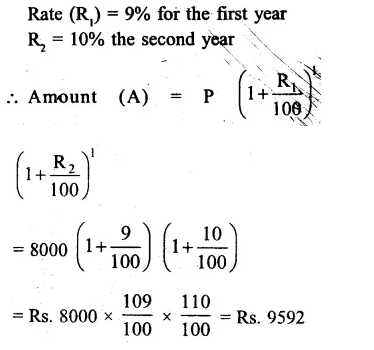Question 8.
Solution:
Principal (p) = Rs. 1, 25,000
Rate of interest (r) = 8% p.a.
Period (n) = 3 years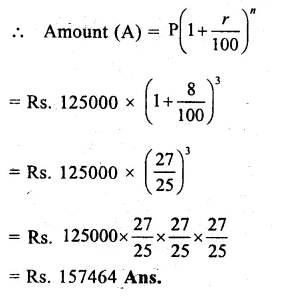Question 9.
Solution:
Price of a buffalo (P) = Rs. 11000
Rate of interest (R) = 10% p.a.
Period (n) = 3 years
Price of buffalo at presentQuestion 10.
Solution:
Amount of loan taken (P)
= Rs. 18000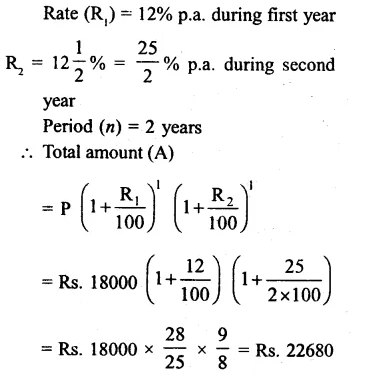Question 11.
Solution:
Amount borrowed from Bank (P) = Rs. 24000
Rate (R) = 10% p.a.
Period (n) = 2 years 3 months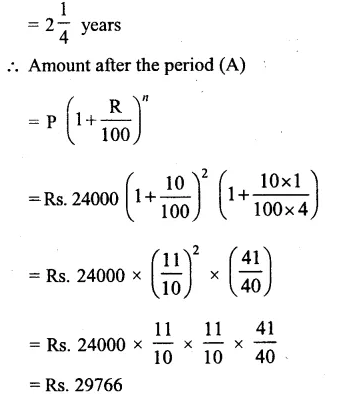Question 12.
Solution:
In case of Abhay,
Principal (p) = Rs. 16000Question 13.
Solution:
Simple interest (S.I.) = Rs. 2400
Rate (R) = 8% p.a.
Period (T) = 2 years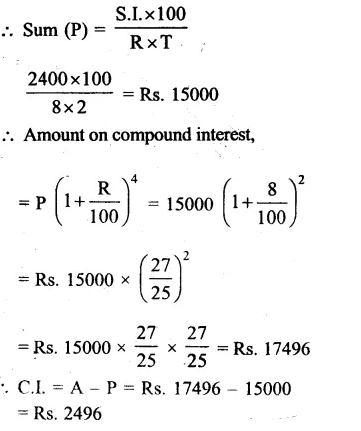Question 14.
Solution:
Difference between C.I. and S.I.
= Rs. 90
Rate (R) = 6% p.a.
Period (n) = 2 years
Let principal (P) = Rs. 100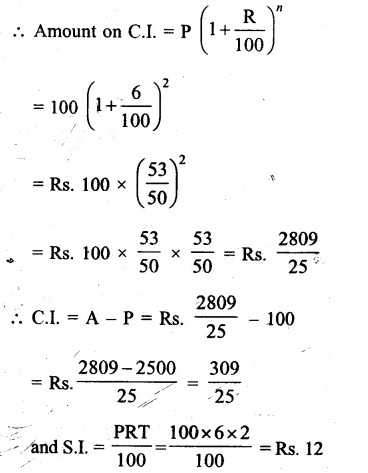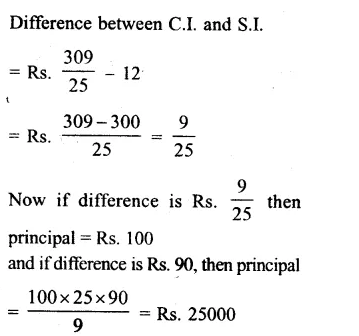Question 15.
Solution:
Let sum (p) = Rs. 100
Rate (r) 10% p.a.
Period (t) = 3 years.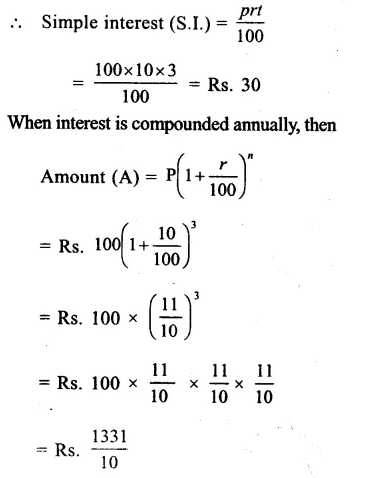Question 16.
Solution:
Amount (A) = Rs. 10240
Rate (r) = $$6\frac { 2 }{ 3 }$$% = $$\\ \frac { 20 }{ 3 }$$% p.a.
Period (n) = 2 years
Let sum = P, then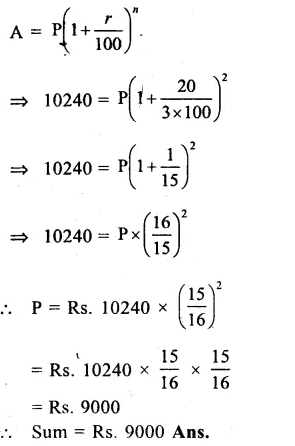Question 17.
Solution:
Amount (A) = Rs. 21296
Rate (r) = 10% p.a.
Period (n) = 3 years.
Let P be the sum, Then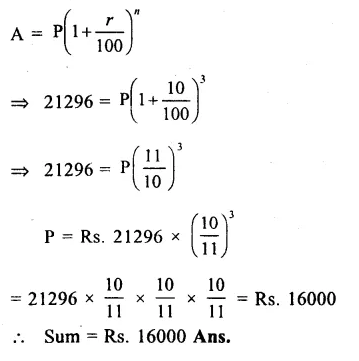Question 18.
Solution:
Principal (P) = 4000
Amount (A) = Rs. 4410
Period (n) = 2 years
Let r be the rate per cent per annum
We know that,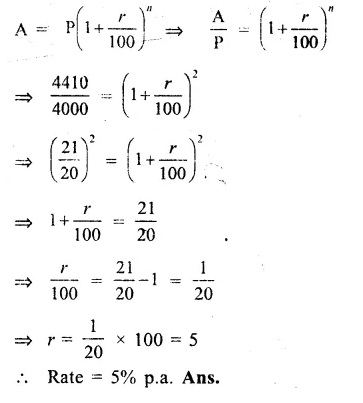Question 19.
Solution:
Principal (P) = Rs. 640
Amount (A) = Rs. 774.40
Period (n) = 2 years
Let r be the rate per cent per annum.
We know that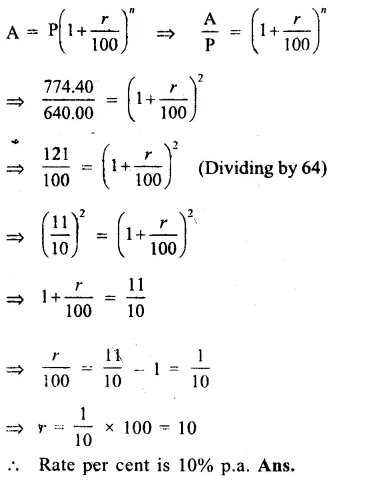Question 20.
Solution:
Principal (P) = Rs. 1800
Amount (A) = Rs. 2178
Rate (r) = 10% p.a.
Let n be the number of years,
We know thatQuestion 21.
Solution:
Principal (P) = Rs. 6250
Amount (A) = Rs. 7290
Rate (R) = 8% p.a.
Let n be the time, then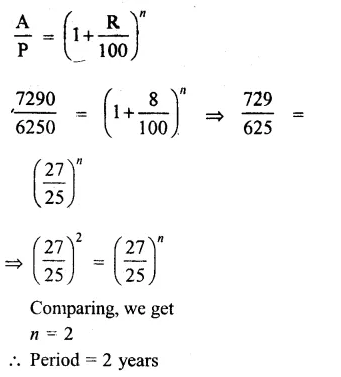Question 22.
Solution:
Present population (P) = 125000
Rate of increasing (R) = 2% p.a.
Period (n) = 3 years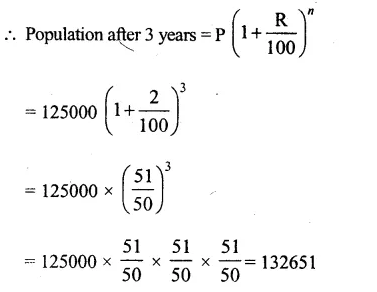Question 23.
Solution:
3 years ago, the population was = 50000
Rate of increase successively (r1, r2, r3) = 4%, 5% and 3% p.a.
Period (n) = 3 years
Present PopulationQuestion 24.
Solution:
Population of a city in 2013 = 120000
Increase in next year = 6%
and decrease in the following year = 5%
Population in 2015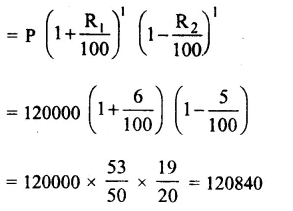Question 25.
Solution:
Initially bacteria = 500000
Increase in bacteria = 2% per hour
Period (n) = 2 hours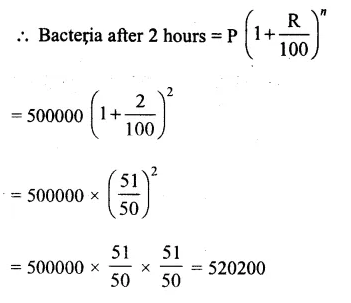Question 26.
Solution:
Growth of bacteria in a culture (R1) = 10% in first hour
Decrease in next hour (R2) = 10%
Increase in the third hour (R3) = 10%
Bacteria in the beginning = 20000
Bacteria after 3 hours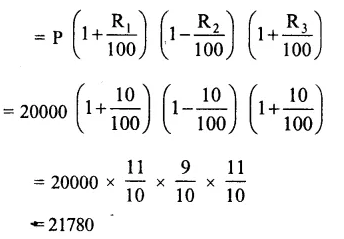Question 27.
Solution:
Value of machine (P) = Rs. 625000
Rate of depreciation (R) = 8% p.a.
Period (n) = 2 yearsQuestion 28.
Solution:
Value of scooter (P) = Rs. 56000
Rate of depreciation (R) = 10% p.a.
Period = 3 years
Value of scooter after 3 yearsQuestion 29.
Solution:
Cost of car = Rs. 34800
Rate of depreciation (R1) = 10% p.a. for first year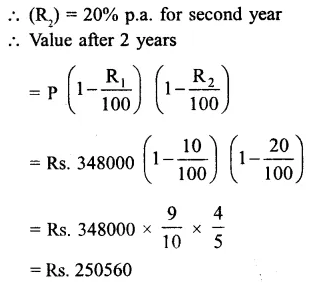Question 30.
Solution:
Rate of depreciation (R) = 10% p.a.
Period (n) = 3 years
Present value (A) = Rs. 291600
Value of machine 3 years agoHope given RS Aggarwal Solutions Class 8 Chapter 11 Compound Interest Ex 11B are helpful to complete your math homework.

If you have any doubts, please comment below. Learn Insta try to provide online math tutoring for you.